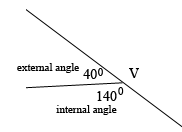SEARCH HOMEMath Central Quandaries & QueriesQuestion from Shriya, a student: All the angles of a polygon are either 155° or 140° There are twice as many angles of 155 °or 140°. Find the number of sides of the polygonHi,

I can help get you started.

Look at one of the vertices $V$ where the internal angle measures $140^{0}.$ The external angle then measures $180^0 - 140^0 = 40^{0}.$What is the measure of the external angle at a vertex where the internal angle measures $155^{0}?$

Now look at Walter's note about the sum of the measures of the external angles of a convex polygon.

Write back if you need more assistance,
PennyMath Central is supported by the University of Regina and the Imperial Oil Foundation.